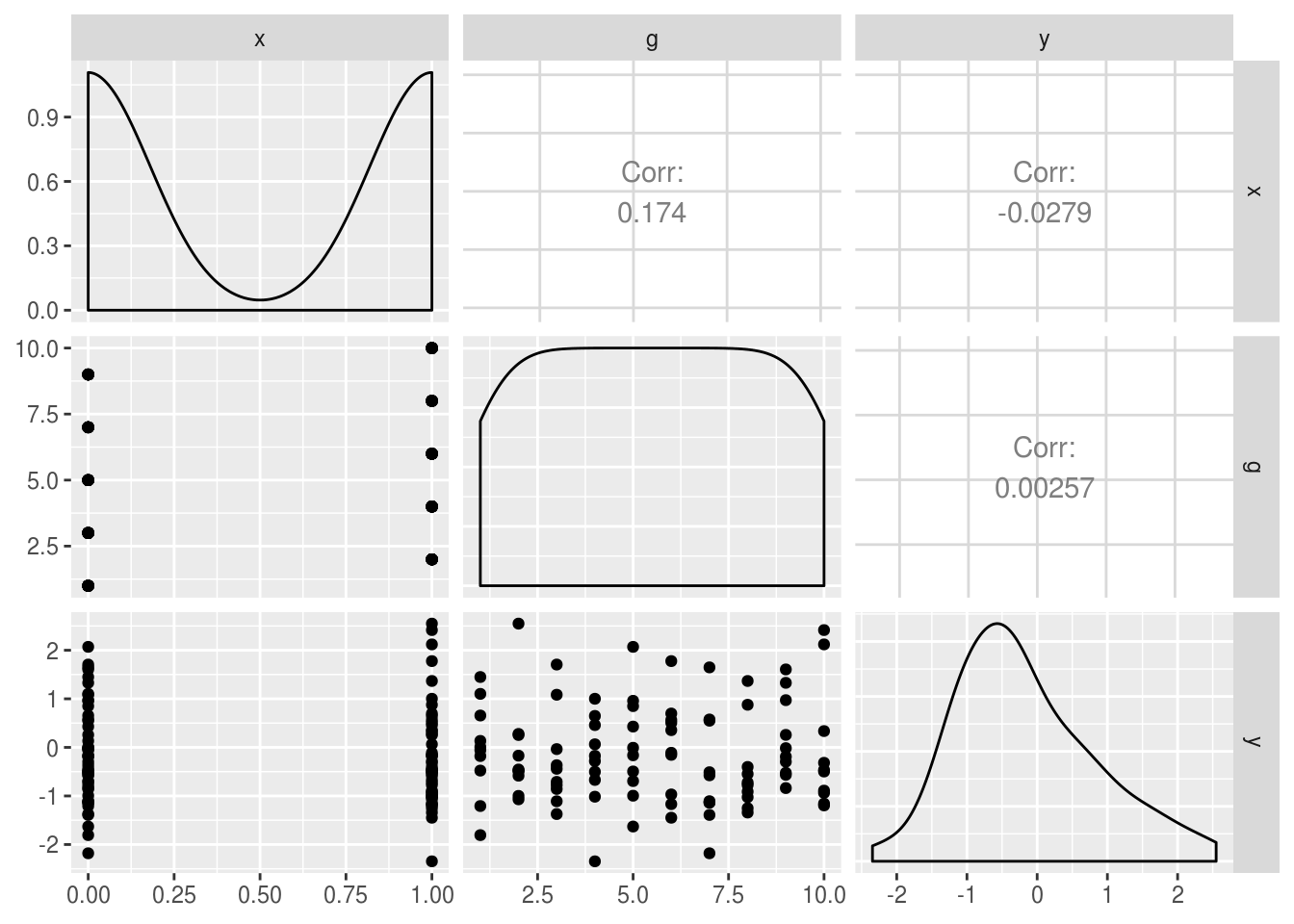# 13 Power analysis

### For most inferential statistics

If you want to do power analysis for a standard statistical test, e.g. t-tests, chi2 or Anova, the pwr:: package is what you need. This guide has a good walkthrough.

### For multilevel or generalised linear models

If you’d like to run power analyses for linear mixed models (multilevel models) then you need the simr:: package. It has some neat features for calculating power by simulating data and results from a model you specify.

To take a simple example, let’s fabricate some data where outcomes y are nested within groups g, and where there is a covariate of interest x. That is, we are interested in estimating our power to detect an effect of x.

Note though that in this simulated data, we create outcomes (y) with no relationship to x, and no clustering by g at this stage (i.e. y, x and g are uncorrelated).

set.seed(1234)
simulated.df <- data_frame(
x = rep(0:1, 50),
g = rep(1:10, 10),
y = rnorm(100)
)
Warning: data_frame() is deprecated, use tibble().
This warning is displayed once per session.
GGally::ggpairs(simulated.df)
Registered S3 method overwritten by 'GGally':
method from
+.gg   ggplot2To use simr:: we first run our ‘model of interest’. In this case it’s a random-intercepts multilevel model. We can verify x is unrelated to outcome with lmerTest::anova:

library(lmerTest)

Attaching package: 'lmerTest'
The following object is masked from 'package:lme4':

lmer
The following object is masked from 'package:stats':

step
model.of.interest <- lmer(y ~ x + (1|g), data=simulated.df)
boundary (singular) fit: see ?isSingular
anova(model.of.interest)
Type III Analysis of Variance Table with Satterthwaite's method
Sum Sq  Mean Sq NumDF DenDF F value Pr(>F)
x 0.077811 0.077811     1    98  0.0764 0.7828

The next step is to modify our saved model of interest. We tweak the fitted parameters to represent our predicted values for effect sizes, variances, and covariances.

First, let’s change the ‘true’ effect of x to be 0.2:

fixef(model.of.interest)['x'] <- .2

We now use the powerSim function to use our tweaked model to:

• create a dataset using the parameters of our model (i.e. make random draws of y which relate to g and x as specified in the model summary).
• re-run lmer on this simulated data.
• repeat this hundreds or thousands of times
• count how many times (i.e., for what proportion) we get a significant p value
power.sim
Power for predictor 'x', (95% confidence interval):
11.00% ( 5.62, 18.83)

Test: unknown test
Effect size for x is 0.20

Based on 100 simulations, (1 warning, 0 errors)
alpha = 0.05, nrow = 100

Time elapsed: 0 h 0 m 11 s

Our observed power (proportion of times we get a significant p value) is very low here, so we might want increase our hypothesised effect of x, for example to see what power we have to detect an effect of x = .8:

fixef(model.of.interest)['x'] <- .8
power.sim
Power for predictor 'x', (95% confidence interval):
95.00% (88.72, 98.36)

Test: unknown test
Effect size for x is 0.80

Based on 100 simulations, (0 warnings, 0 errors)
alpha = 0.05, nrow = 100

Time elapsed: 0 h 0 m 11 s

We might also want to set one of the variance parameters of our model to represent clustering within-g. First we can use VarCorr() to check the variance parameters of the model we just ran:

VarCorr(model.of.interest)
Groups   Name        Std.Dev.
g        (Intercept) 0.0000
Residual             1.0091  

And we could simulate increasing the variance parameter for g to 0.5:

VarCorr(model.of.interest)['g'] <- .5
power.sim
Power for predictor 'x', (95% confidence interval):
33.00% (23.92, 43.12)

Test: unknown test
Effect size for x is 0.80

Based on 100 simulations, (0 warnings, 0 errors)
alpha = 0.05, nrow = 100

Time elapsed: 0 h 0 m 11 s

Because the amount of clustering in our data has increased our statistical power has gone down. This is because, when clustering is present, each new observation (row) in the dataset provides less new information to estimate our treatment effect. Note that in this example we increased the variance associated with g by quite a lot: setting the variance of g to 0.5 equates to an ICC for g of .33 (because .5 / (.5 + 1) = .33; see the section on calculating ICCs and VPCs)

For more details of simr see: http://onlinelibrary.wiley.com/doi/10.1111/2041-210X.12504/full

Note that for real applications you would want to set nsim to something reasonably large. Certainly at least 1000 simulations, and perhaps up to ~5000.